# Given the following data for each of the salts answer the questions below: KCINH.NO, Literature AH,...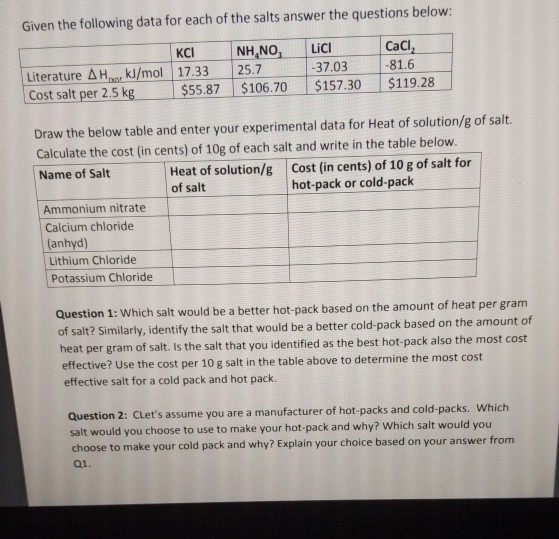Given the following data for each of the salts answer the questions below: KCINH.NO, Literature AH, kJ/mol 17.33 25.7 Cost salt per 2.5 kg \$55.87 \$106.70 LICI -37.03 \$157.30 CaCl, -81.6 \$119.28 Draw the below table and enter your experimental data for Heat of solution/g of salt. Calculate the cost in cents) of 10g of each salt and write in the table below. Name of Salt Heat of solution/g Cost (in cents) of 10 g of salt for of salt hot-pack or cold-pack Ammonium nitrate Calcium chloride (anhyd) Lithium Chloride Potassium Chloride Question 1: Which salt would be a better hot-pack based on the amount of heat per gram It? Similarly, identify the salt that would be a better cold-pack based on the amount of heat per gram of salt. Is the salt that you identified as the best hot-pack also the most cost effective? Use the cost per 10 g salt in the table above to determine the most cost effective salt for a cold pack and hot pack. Question 2: Clet's assume you are a manufacturer of hot-packs and cold-packs. Which salt would you choose to use to make your hot-pack and why? Which salt would you choose to make your cold pack and why? Explain your choice based on your answer from 01.

The heat transfer, or change in enthalpy in a reaction (qrxn), is related to the mass of the solution (m), the specific heat capacity of the solution (c), and the temperature change

(ΔΤ = Τfinal — Τinitial).

qrxn= – (m × c × ΔΤ)

The specific heat capacity of a substance is the amount of energy required to raise 1 g of the substance 1° C. The specific heat capacity of water is 4.186 J / (° C × g). In experiments conducted in aqueous solution, the specific heat capacity of water is generally used.

HOT PACK and COLD PACK

Many instant hot and cold packs function by dissolving a salt into water. As the salt disassociates, heat is either released in an exothermic reaction or absorbed in an endothermic reaction. Commercial instant cold packs typically use either ammonium nitrate or urea as their salt component; hot packs often use magnesium sulfate or calcium chloride. These reactions happen in a similar manner. When the salt is dissolved in water, the ionic bonds of the salt separate. This process requires energy, which is obtained from the surroundings.

The ions then form bonds with the water, a process that releases energy. If more energy is released than taken in, then the process is exothermic, making the solution feel warmer. If more energy is taken in than released, then the process is endothermic, making the solution feel cooler.

So we must Calculate the heat absorbed by the water for each mass of the chemical salt and the heat absorbed by the calorimeter for each mass of chemical salt. The specific heat of water is 4.186 J/° C × g.

qω = – [cω × mω × ΔΤ]

Since the experimental values are not provided so use this concept and the outline to figure it out.

#### Earn Coin

Coins can be redeemed for fabulous gifts.

Similar Homework Help Questions
• ### 5g CaCl2 100.74 10g CaCl2 100.74 15g CaCl2 100.74 5g NH.CI 100.74 5g Mass of water...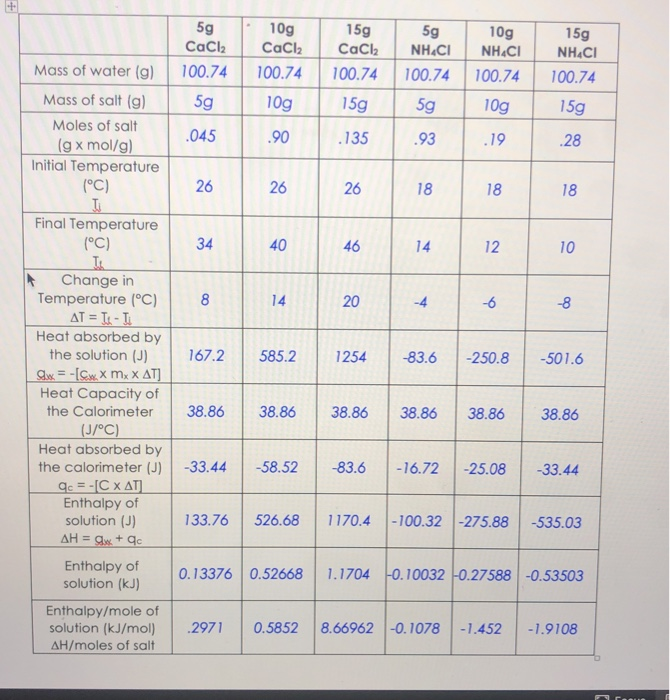5g CaCl2 100.74 10g CaCl2 100.74 15g CaCl2 100.74 5g NH.CI 100.74 5g Mass of water (g) 10g NH.CI 100.74 10g 15g NH4Cl 100.74 5g 15g Mass of salt (g) Moles of salt (g x mol/g) Initial Temperature (°C) .045 .90 .135 26 26 26 18 18 Final Temperature (°C) 46 14 12 10 8 167.2 585.2 1254 -83.6 -250.8 -501.6 Change in Temperature (°C) AT = T- Heat absorbed by the solution (J) Slx = -(Sw.X mxX AT] Heat...

• ### Given the following estimated demand equation Answer the following questions “From the data for 46 States...

Given the following estimated demand equation Answer the following questions “From the data for 46 States in the United States for 1002, the following Regression Equation was estimated: Ln C =             6.30 – 1.39 LnP + 0.67 LnY T- Stats:          (0.91) (- 2.45)     ( 0.45)                      R2 =0.78 Where C = Cigarette consumption packs per year             P = real price per pack             Y = real disposable income per capita            a). What is the elasticity of demand for...

• ### 2 Net ionic equation for ttradon of iron with potassium permanganate is: ([email protected] each) S MnO...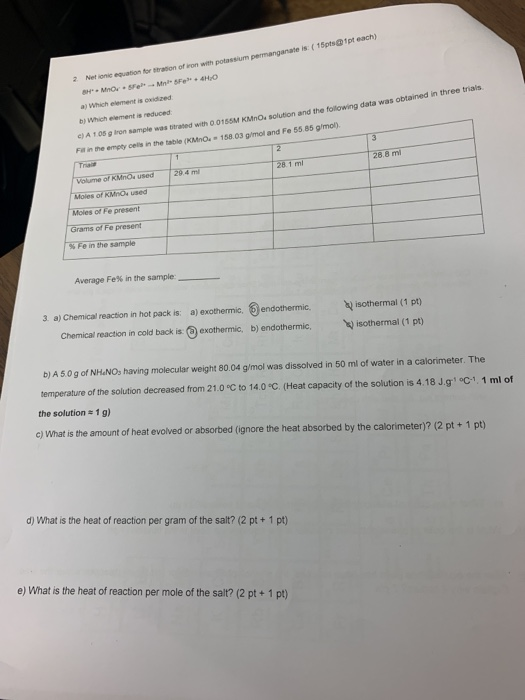2 Net ionic equation for ttradon of iron with potassium permanganate is: ([email protected] each) S MnO SFel Mn SFe 4H0 a) Which element is oxidized e) A 1.08 g iron sample was titrated with O 0155M KMnOs soluticn and the folowing data was obtained in three trials F in the empty cells in the table (KMnO, 158.03 g/mol and Fe 55 85 g/mol). b Which element is reduced 3 2 Trait 1 28.8 ml 28 1 ml 294 m Volume...

• ### A table of enthalpies of solvation in water is given below for various substances at 298...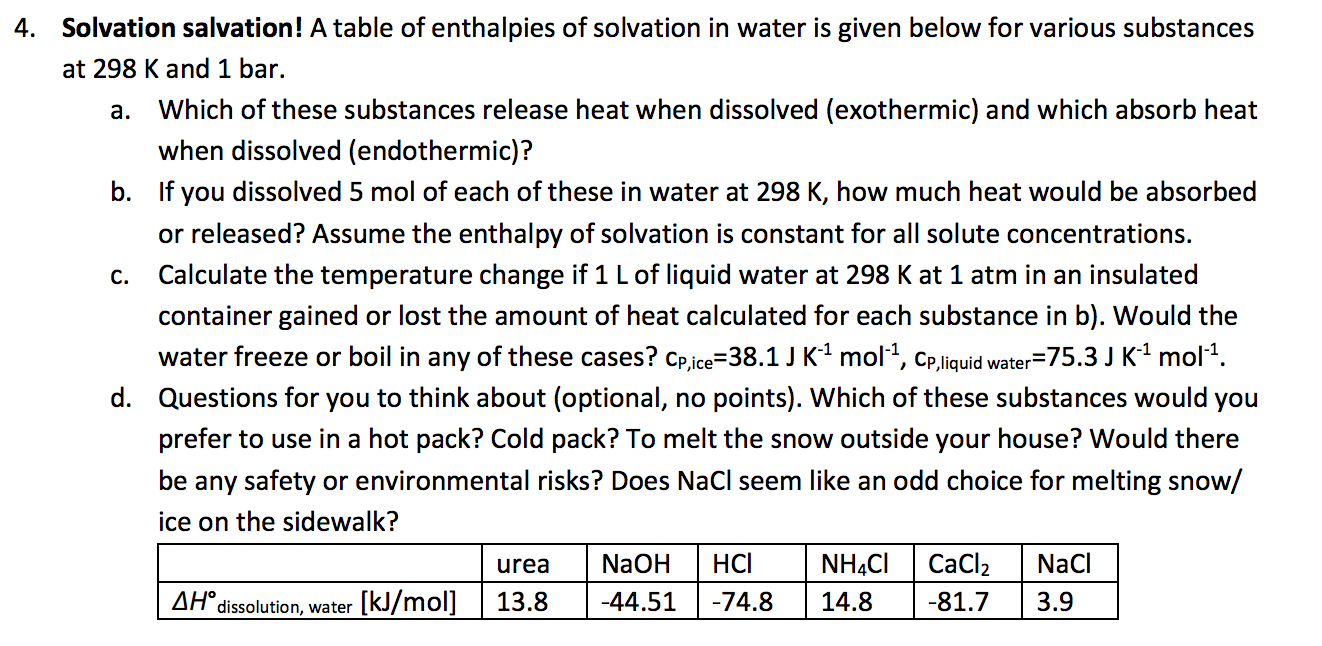A table of enthalpies of solvation in water is given below for various substances at 298 K and 1 bar. Which of these substances release heat when dissolved (exothermic) and which absorb heat when dissolved (endothermic)? If you dissolved 5 mol of each of these in water at 298 K, how much heat would be absorbed or released? Assume the enthalpy of solvation is constant for all solute concentrations. Calculate the temperature change if 1 L of liquid water at...

• ### 2) (2 pts) If you dissolve 10.0 g of compound X in 100.0 mL of distilled...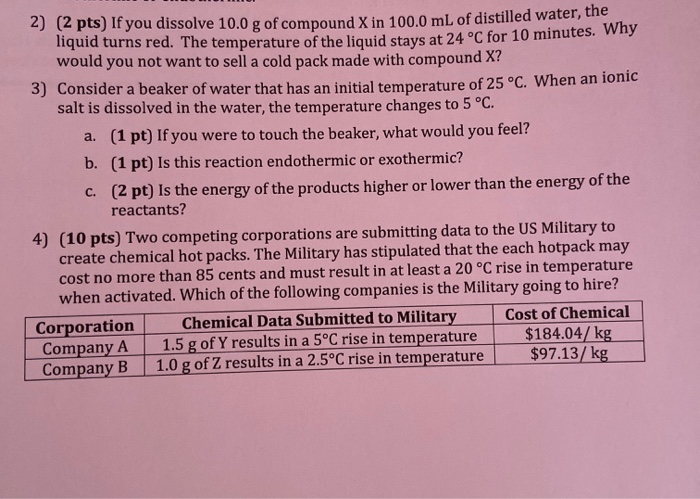2) (2 pts) If you dissolve 10.0 g of compound X in 100.0 mL of distilled water, the liquid turns red. The temperature of the liquid stays at 24 °C for 10 minutes. why would you not want to sell a cold pack made with compound X? 3) Consider a beaker of water that has an initial temperature of 25 °C. When an ionic salt is dissolved in the water, the temperature changes to 5 °C. a. (1 pt) If...

• ### 2) (2 pts) If you dissolve 10.0 g of compound X in 100.0 mL of distilled water, the liquid turns red. The temperatu...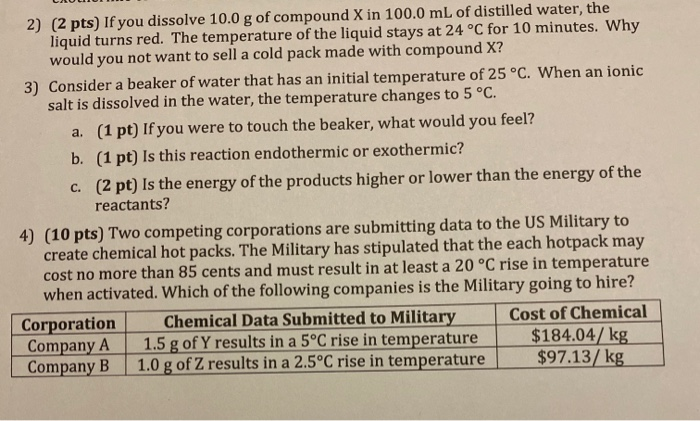2) (2 pts) If you dissolve 10.0 g of compound X in 100.0 mL of distilled water, the liquid turns red. The temperature of the liquid stays at 24 °C for 10 minutes. Why would you not want to sell a cold pack made with compound X? 3) Consider a beaker of water that has an initial temperature of 25 °C. When an ionic salt is dissolved in the water, the temperature changes to 5 °C. a. (1 pt) If...

• ### The following data was collected after running a calorimetric experiment using three salts. The data collected...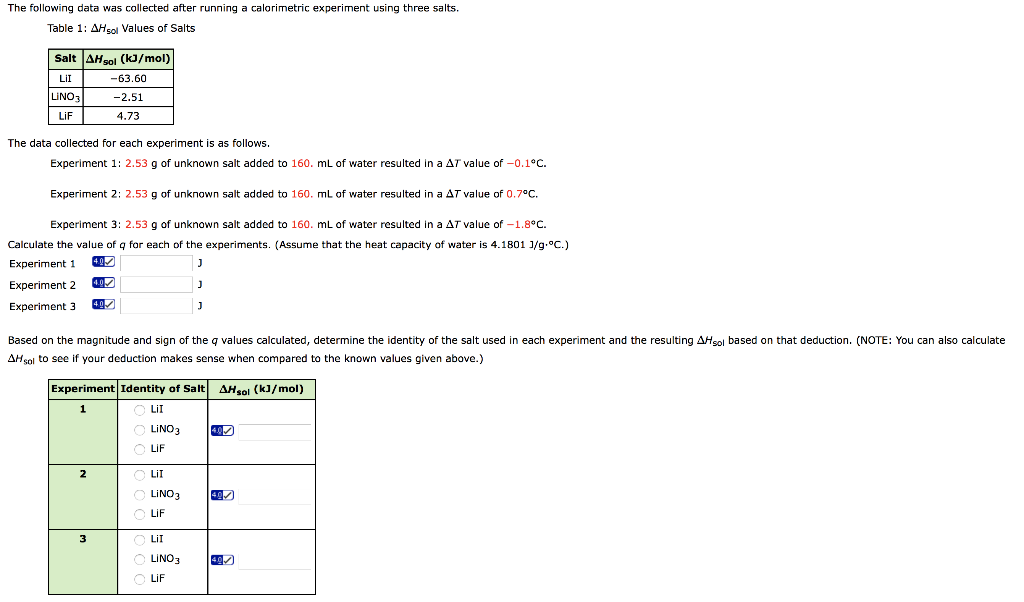The following data was collected after running a calorimetric experiment using three salts. The data collected for each experiment is as follows. Experiment 1: 2.53 g of unknown salt added to 160. mL of water resulted in a ΔT value of −0.1°C. Experiment 2: 2.53 g of unknown salt added to 160. mL of water resulted in a ΔT value of 0.7°C. Experiment 3: 2.53 g of unknown salt added to 160. mL of water resulted in a ΔT value...

• ### a and b pls 3. Answer the following questions regarding the neutralization of HCl using NaOH: a. If the volume o...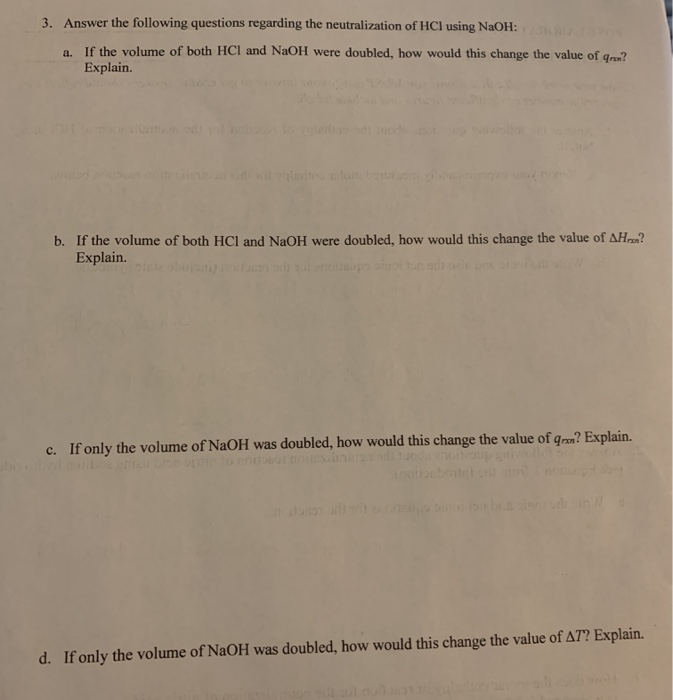a and b pls 3. Answer the following questions regarding the neutralization of HCl using NaOH: a. If the volume of both HCl and NaOH were doubled, how would this change the value of nu? Explain. b. If the volume of both HCl and NaOH were doubled, how would this change the value of AHan? Explain. c. If only the volume of NaOH was doubled, how would this change the value of qrx? Explain. d. If only the volume of...

• ### 2. Answer the following questions about the neutralization reaction of nitric acid using sodium hydroxide (see...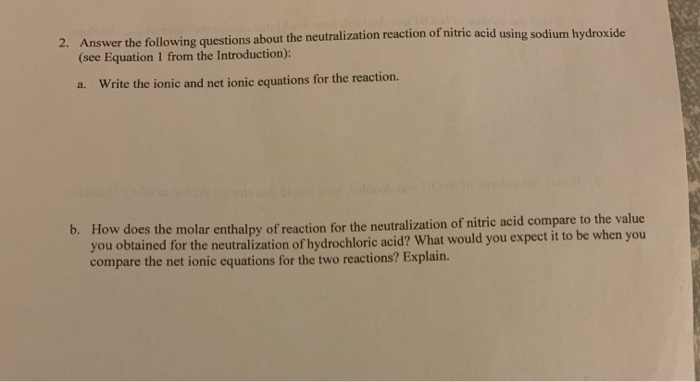2. Answer the following questions about the neutralization reaction of nitric acid using sodium hydroxide (see Equation 1 from the Introduction): a. Write the ionic and net ionic equations for the reaction. b. How does the molar enthalpy of reaction for the neutralization of nitric acid compare to the value you obtained for the neutralization of hydrochloric acid? What would you expect it to be when you compare the net ionic equations for the two reactions? Explain. livier, using Eos...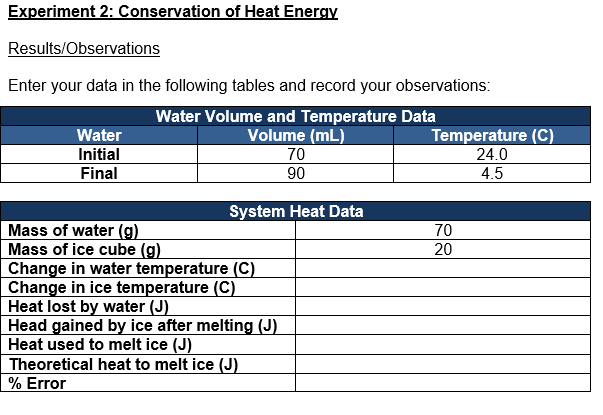Please help me answer the following questions given the data that I obtained, and please type your answer showing step by step calculations and formulas please Experiment 2: Conservation of Heat Energy – Analysis and Discussion Based on your experimental results, please answer the following questions: Given the initial and final volumes of the experiment, determine the initial mass of the water and the mass of the ice cube. Show your work. Record your answer in the table above. Given...